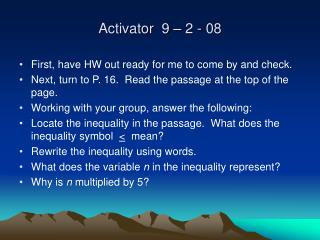DownloadDownload PresentationActivator 9 – 2 - 08

# Activator 9 – 2 - 08

Télécharger la présentation## Activator 9 – 2 - 08

- - - - - - - - - - - - - - - - - - - - - - - - - - - E N D - - - - - - - - - - - - - - - - - - - - - - - - - - -
##### Presentation Transcript

1. Activator 9 – 2 - 08 • First, have HW out ready for me to come by and check. • Next, turn to P. 16. Read the passage at the top of the page. • Working with your group, answer the following: • Locate the inequality in the passage. What does the inequality symbol < mean? • Rewrite the inequality using words. • What does the variable n in the inequality represent? • Why is n multiplied by 5?

2. How are open sentence equations and inequalities solved? • Open sentence – a mathematical sentence with one or more variables. • The process of finding a value that makes an open sentence true is called solving the opensentence. • A set of numbers from which replacements for a variable may be chosen is called a replacement set. • The solution set is the set of elements that makes the open sentence true.

3. Equations and Inequalities • Equations • Has an equal sign. - Is an open math sentence. • Has one solution. • Can be solved using order of operations or by using a replacement set. • Inequality • Has an inequality symbol: <, >, <, > • Is an open math sentence. • Has infinite number of solutions. • Can be solved using order of operations or by using a replacement set.

4. To use a replacement set to solve an equation or inequality: • Find the solution set for each equation if the replacement set is (2, 3, 4, 5, 6). • A. 4a + 7 = 23 • B. 3(8 – b) = 6 *Hint, begin by using theDistributive Property. • Find the solution set for the inequality if the replacement set is (20, 21, 22, 23, 24) • C. Z + 11 > 32 *Hint, inequalities have more than one solution.

5. To use order of operations to find the solution set of an equation or inequality: • You can also solve an equation by applying the order of operations. • Solve the following: • 5(8 – 2) • 18 – (5 – 3)^3 = k Hint, the fraction bar is a grouping symbol.

6. Solve the following: • A four-wheel-drive tour of Canyon de Chelly National Monument in Arizona cost \$45 for the first vehicle and \$15 dollars for each additional vehicle. How many vehicles can the Velo family take on the tour if they want to spend no more than \$100? • First think – do I set up an equation or an inequality? Look for key words to decide. • Set up and solve. Show all work.

7. Summarizer • Get out a clean sheet of paper. Turn landscape style. • Create a double bubble map comparing equations and inequalities.